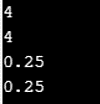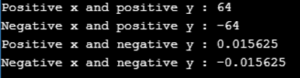# How to Implement Power Function in PythonIn today’s modern times, Python is undoubtedly one of the most prominent and popular programming languages out there. Python comes with a host of different functions each built specifically to add more versatility to the interface than before. One such function of the Python ecosystem is the power function, also represented as the pow(). In this article, we will discuss the Power function in Python.

## Introduction to the Power Function in Python?

The power function in Python can be used when one needs to derive the power of variable x to the variable y. If in a particular situation, the user includes a third variable that is z into the equation, then the pow() function returns x to the power of y, modulus of z. This in mathematical terms, looks something like this, pow(x, y) % z.

The syntax for Power function:

`pow(x, y[, z])`

If you are computing the pow (x,y) then the output will be, x**y.

## Parameters of the Power Function

Now that you are accustomed to the basics of the Power Function in Python, let us explore the various parameters that are involved in the creation of the same.

When using the power method three parameters are taken into account.

1. X: x denotes the number which is to be powered.

2. Y: y denotes the number which is to be powered with x.

3. Z: z is an optional variable and is used to derive the modulus of power x and y.

Parameter Cases for Power method

1. X: X can either be a non-negative integer or a negative integer whenever it is being used.

2. Y: Y can also be a non-negative integer or a negative integer when used in the equation.

3. Z: In most cases, z is an optional variable and may or may not be present.

## Return Values for Pow()

Dependent upon the situation it is used at, the power method returns several different variables. Some of the most significant ones are as mentioned below.

 X Y Z Return Value Non-negative Integer Non-negative Integer N/A Integer Non-negative Integer Negative Integer N/A Float Negative Integer Non-negative Integer N/A Integer Negative Integer Negative Integer N/A Integer Negative/Non-negative Integer Non-negative Integer Negative/Non-negative Integer Integer

## Example Code

• Example 1:
```# positive x, positive y (x**y)
print(pow(2, 2))

# negative x, positive y
print(pow(-2, 2))

# positive x, negative y (x**-y)
print(pow(2, -2))

# negative x, negative y
print(pow(-2, -2))```

Output:• Example 2:
```x = 7
y = 2
z = 5

print(pow(x, y, z))```

Output:

`4`

• Example 3:
```# Python code to demonstrate naive method
# to compute power
n = 1
for i in range(1,5):
n=3*n

print ("The value of 3**4 is : ",end="")
print (n)```

Output:

`The value of 3**4 is : 81`

• Example 4:
```# Python code to demonstrate pow()
# version 1

print ("The value of 3**4 is : ",end="")

# Returns 81
print (pow(3,4))```

Output:

`The value of 3**4 is : 81.0`

• Example 5:
```# Python code to demonstrate pow()
# version 2

print ("The value of (3**4) % 10 is : ",end="")

# Returns 81%10
# Returns 1
print (pow(3,4,10))```

Output:The power function in Python, when used correctly can eliminate a lot of stress and confusion. We hope that from this article you have learned how to use the power function in Python correctly and thus will make use of the same in your day to day programming.

With this, we come to an end of this Power Function in Python article. To get in-depth knowledge on Python along with its various applications, you can enroll now for live Python course training with 24/7 support and lifetime access.

Got a question for us? Mention them in the comments section of this article and we will get back to you.

Upcoming Batches For Python Certification Training Course
Course NameDate
Python Certification Training Course

Class Starts on 25th February,2023

25th February

SAT&SUN (Weekend Batch)
View Details
Python Certification Training Course

Class Starts on 25th March,2023

25th March

SAT&SUN (Weekend Batch)
View DetailsREGISTER FOR FREE WEBINARThank you for registering Join Edureka Meetup community for 100+ Free Webinars each month

### Subscribe to our Newsletter, and get personalized recommendations.#### How to Implement Power Function in Python

edureka.co